# Food Technology: Sizing a Food Can Using Calculus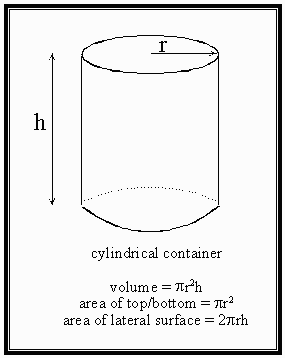## Problem:

A closed top cylindrical container is to hold a volume of 1.875 litres. The material used for the top and bottom parts of the container costs twice as much per unit area as the material used for the cylindrical tube. Find the dimensions of the most economical container.

## Solution:

We need to find the dimensions of this container which minimize the total cost of material. Maximization/minimization problems of this type are all solved using the following steps.

### Step 1:

Write down a formula for the quantity that is to be maximized or minimized: here the Cost, C, of materials, required. We need to define r to be the radius of the circular cross section of the container, and h to be its vertical height. Further, let k stand for the cost per square centimeter of the material used in fabricating the cylindrical tube. Then the material used for the circular top and bottom of the container will cost 2k per square centimeter. Thus,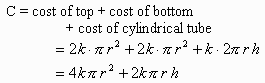We have two independent variables here, but one can be eliminated using the requirement that the container hold 1.875 litres. Equating the formula for the volume of the container to this value gives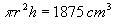where we have converted the given volume to its equivalent in cm3 to facilitate balancing of units later. Thus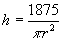in units of cm. Substituting this into our formula so far for C gives finally,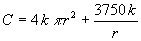Since k is a constant (which does not need to be known to answer this question), we have now successfully expressed C as a function of the single independent variable, r.

### Step 2

The maximum or minimum of a function will occur either for values of the independent variable where the first derivative equals zero, or at the extreme possible values of the independent variable (in this case r = 0 or r =∞ both give C = ∞ , so these options can be ignored). Thus, differentiating,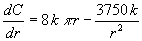Setting this to zero gives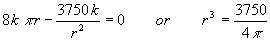(The k has cancelled out.) Taking the cube root gives to two decimal places, r = 4.56 cm, and substituting into the formula for h gives 28.67 cm.

### Step 3

There is just one set of dimensions that may give a minimum cost container. We need to provide an argument, all the same, that these dimensions do give a minimum cost. (After all, we may have improperly stated our goal, and so this single solution could give the most expensive, or maximum cost, container). The easiest way to argue this point is to note that the second derivative of the cost,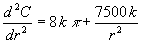is positive for all positive values of r, and so is certainly positive for r = 4.56 cm. This confirms that we have a minimum value of C here.

Thus, the container with the lowest cost of materials should have a radius of 4.56 cm, and a height of 28.67 cm.

## Possible Variations

1. Find the dimensions of the cylindrical container with a 1.875 litre capacity which will minimize the total surface area of the container.
2. Assume that the circular top and bottom of the container must be cut from square pieces of material measuring 2r on a side, with the extra bits of material being considered waste. What dimensions of the 1.875 litre capacity container would now lead to use of least material per container?
3. Typically a seam exists vertically down the cylindrical tube, and around the edges of the circular top and bottom of the can. Find the dimensions of the 1.875 litre capacity cylindrical container which minimizes the total length of seams.
4. One could start out with a specified total surface area, say 1200 cm2, and then find the dimensions of the cylindrical container which would have the largest volume capacity.Written by David Sabo, October 6, 1997